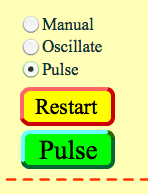# Sources of waves

When we have a single disturbance or event, a pulse is created which propagates away the energy from that event.  A periodic disturbance (or a vibration) would create repeating pulses, or a wave.  We could describe the back and forth motion of an object vibrating in Simple Harmonic Motion by the equation

Y = SIN (ωt)

where y is the amplitude of the vibration, ω is angular frequency, and t is time.The angular frequency is measured in radians per second and is related to frequency by a unit conversion

ω=2πf

where f, frequency is usually measured in Hertz or oscillations per second.  Because the units are in radians, we often use radian mode on our calculators (instead of degree mode).   We could also express this in terms of the Period of time for one oscillation, T.

T = 1/f  and ω=2πf = 2π/T

When this period disturbance or vibration propagates outward, it is not only a function of time, but also of position.Using the below simulation, you can wiggle the wrench on the rope and see the resulting waves propagate outwards.  Set the simulation for NO END so we don’t have to worry about reflections (which we will discuss in good time).

If you set the simulation for PULSE, you will see the pulses traveling outwards.  With OSCILLATE  you will see the waves traveling outward.As you observe these waves you will notice that the position of the rope varies both with position along the rope, x, and time, t.  We can express this with the equation

Y = SIN (kx-ωt)

where k is what we call the wave number and is related to the spacing between pulses or the wavelength.

# Parts of a wave:

Usually when we think of a wave, we might consider a sine wave, where the high points of the wave are called crests, and the low points are called troughs.  The displacement of the wave from its equilibrium position to the maximum point of the crest (or the minimum point of the trough) is called the amplitude, A.   If we are looking at a graph which is a function of displacement versus time, then the difference in time between two crests is called the period.If we are looking at a graph which is a function of displacement versus position , then the difference in time between two crests is called the wavelength, λ .  We mentioned earlier, the wave number k, which is actually the reciprocal of wavelength.

k = 2π/λ# What is moving?

Something to consider when looking at a wave is what is actually propagating forward?

We saw in class a ripple tank where a vibrating stick creates water waves.

Another nice example we saw in class was a wave propagating on a wave machine.  But what actually moves forward?

Another question might be what is being moved? Most waves will temporarily displace something as they travel.   In the case of a water wave, the answer is self explanatory.  Electromagnetic waves is actually electromagnetic fields that propagate, and interact only with charges.  Gravitational waves interact with mass.  Sound waves will compress the medium they travel through.

# Transverse vs Longitudinal Waves

We shouldn’t assume that the disturbance has to be perpendicular to the direction of the propagation. When it is perpendicular, producing crests and troughs, we call it a transverse wave. However, if the disturbance is parallel to the direction of propagation, we call it a longitudinal wave. In this case, instead of crests & troughs, we have compressions are rarefactions as you can see in this video.

Here is a nice simulation with transverse vs longitudinal waves by Professor Duffy in the BU Physics Department.

I want to end with a link to a Phet Simulation where you can play with two-dimension waves such as the ripple tank!